[BACK]Computer Modeling in Engineering & SciencesDOI: 10.32604/cmes.2021.014027

ARTICLE

An Efficient Algorithm Based on Spectrum Migration for High Frame Rate Ultrasound Imaging

1Department of Biomedical Engineering, Hefei University of Technology, Hefei, 230009, China
2Yijishan Hospital of Wannan Medical College, Wuhu, 241001, China
*Corresponding Author: Hu Peng. Email: hpeng@hfut.edu.cn
Received: 29 August 2020; Accepted: 20 October 2020

Abstract: The high frame rate (HFR) imaging technique requires only one emission event for imaging. Therefore, it can achieve ultrafast imaging with frame rates up to the kHz regime, which satisfies the frame rate requirements for imaging moving tissues in scientific research and clinics. Lu’s Fourier migration method is based on a non-diffraction beam to obtain HFR images and can improve computational speed and efficiency. However, in order to obtain high-quality images, Fourier migration needs to make full use of the spectrum of echo signals for imaging, which requires a large number of Fast Fourier Transform (FFT) points and increases the complexity of the hardware when the echo frequency is high. Here, an efficient algorithm using the spectrum migration technique based on the spectrum’s distribution characteristics is proposed to improve the imaging efficiency in HFR imaging. Since the actual echo signal spectrum is of limited bandwidth, low-frequency and high-frequency parts with low-energy have little contribution to the imaging spectrum. We transform the effective part that provides the main energy in the signal spectrum to the imaging spectrum while the ineffective spectrum components are not utilized for imaging. This can significantly reduce the number of Fourier transform points, improve Fourier imaging efficiency, and ensure the imaging quality. The proposed method is evaluated on simulated and experimental datasets. Results demonstrated that the proposed method could achieve equivalent image quality with a reduced point number for FFT compared to the complete spectrum migration. In this paper, it only requires a quarter of the FFT points used in the complete spectrum migration, which can improve the computational efficiency; thus, it is more suitable for real-time data processing. The proposed spectrum migration method has a specific significance for the study and clinical application of HFR imaging.

Keywords: High frame rate imaging; spectrum migration; Fourier migration

1  Introduction

Studies on non-diffraction-based imaging emit a non-diffraction wave-field and have been described since the 1990s [1,2]. The wave-front of non-diffraction waves keeps the same shape when it transmits to an infinite distance. In practice, finite transducers and signals with finite energy are used to approximate the non-diffraction wave; thus, this kind of wave can preserve the characteristics of non-diffraction waves at a certain propagation distance, which is named as limited-diffraction beam (LDB) . The array beam is a non-diffraction beam and was first proposed by Lu . The theory of high frame rate (HFR) imaging based on the array beam was proposed in 1997 [5,6]. A plane wave or an array beam is transmitted to the imaging object in the HFR, and the received echoes are then weighed by a non-diffraction beam with different parameters to estimate the imaging spatial spectrum. Finally, the imaging spatial spectrum is Fourier-transformed to generate the ultrasound image. Since a single transmission can obtain an image, HFR imaging achieves high frame rates. Moreover, the computational load is efficiently reduced because of the utilization of fast Fourier transform (FFT) to process data vs. the delay-and-sum (DAS) method. Peng et al. [7,8] proposed alternative models, in which FFT is used to substitute the weighting process in Lu’s method [5,6]. Therefore, the imaging system is simpler, and the hardware circuit can be more easily implemented. Besides, an equivalent image quality can be obtained versus Lu’s imaging system.

To improve the imaging quality of HFR, two kinds of methods for resolution enhancements in HFR imaging have been proposed by Cheng et al.  and Lu et al. . Cheng et al. , the HFR imaging theory is extended with various explicit transmission schemes are included, e.g., several limited-diffraction array beams and steered plane waves with different angles. This extended theory established the relationship between array beam weighting and Fourier transform of received echo signals; this approach can increase resolution and contrast over a large field of view (FOV). Lu et al. , a modified LDB imaging method with square wave aperture weighting was proposed to reconstruct higher quality images. Delay compensation is not needed with the requirements of one or two transmitters to achieve both transmit and receive focusing. Transmit plane waves with different angles or LDB with different parameters can enlarge the FOV and broaden the range of the frequency spectrum. The ultrasonic echo signal spectrum is transformed to the frequency spectrum using plane waves with different angles or LDB with different parameters. Simulation and experiment have both indicated that the methods could effectively improve the imaging resolution and further reduce the influence of noise on imaging performance. However, the frame rate is reduced, and the computational load is somewhat increased due to the requirement of several transmit events for image generation. Chen et al.  described this process as Fourier migration.

In the study of Chen et al. , the influence of plane waves with different angles or array beams with different parameters on the migration of frequency spectrum was discussed. Furthermore, the influence of different shapes of frequency spectrum after migration on the imaging quality was also discussed. The proposed theoretical model can accurately estimate the spectrum distribution and the point spread function (PSF) shape and can optimize beam forming and post processing in plane-wave-based ultrasound imaging. Although the literature  discusses the relationship between signal spectrum and imaging spectrum and PSF, it does not consider the contribution of effective components in the signal spectrum to imaging spectrum. The imaging spectrum is established in the entire echo signal spectrum; thus, the effective components of the echo signal spectrum are not effectively used, and the efficiency of the imaging algorithm should be further improved.

Zhao et al.  proposed an improved imaging method by combining two different imaging methods, i.e., DAS and Fourier beam forming. The combination of DAS and Fourier beam forming shows the effectiveness in reducing side lobes in DAS and Fourier beam forming thus improving the image quality. Although the proposed method can improve the imaging quality to some extent, DAS and Fourier beam forming methods must be performed on echo signals individually; thus, the complexity of imaging system and computational load are significantly increased.

In the HFR imaging system based on Fourier transform, FFT technique is a core part to realize HFR imaging. Although many studies are developed to improve the quality of HFR imaging, there are few researches aim to reduce the number of FFT points and improve the efficiency of the FFT operation. Since the spectrum of practical signals has a limited bandwidth, not all frequency points in the spectrum are needed to calculate FFT when transforming the echo signal spectrum to the imaging spectrum in Fourier migration. This paper proposes an imaging method using a spectrum migration technique that ignores the low-frequency and high-frequency parts with low-energy to improve the computational efficiency of FFT. The imaging spectrum is migrated from the high-frequency region to the low-frequency region so that the signal spectrum can be fully utilized. This method only migrates the most concentrated part of the spectrum energy in the signal to the imaging spectrum area, thus it ensures image quality and reduces the number of FFT points. The results indicate that the spectrum migration method effectively reduces the number of FFT points and improve the FFT transformation efficiency. It also reduces the influence of side lobes on imaging quality and improves imaging quality in resolution and contrast.

2  Imaging Algorithms

2.1 The Fourier Migration Algorithm Based on an Angular Spectrum Propagation Principle

First, the acoustic field of the impulse plane waveis transmitted by the transducer, and the acoustic signal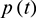is obtained in a plane z = zj. Then, the frequency spectrum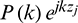of the signal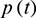can then be obtained after FFT, where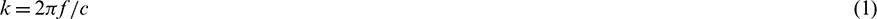The echo signals received from scattering points in the plane z = zj can be expressed as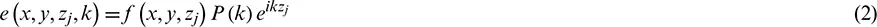where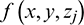is the reflection coefficient function of the scattering point located at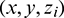. After FFT, the expression of Eq. (2) in angular spectrum domainis obtained as follows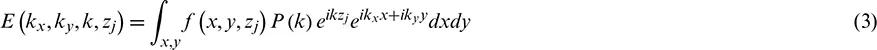Due to the reflection of scattering targets, echoes represented by Eq. (2) are received by the transducer. Based on the theory of angular spectrum propagation, echo signals can be represented asIn reality, echo signals are received from many planes in the acoustic field, and thus the received signal should be the integral of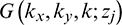in all planes, i.e.,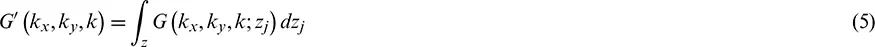The received signal, after a simple mathematical processing using Eqs. (4)(6), can be further expressed as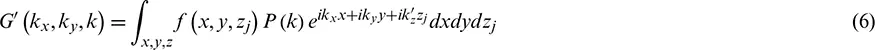where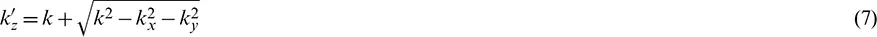Thein Eq. (6) is irrelevant to the integral and can be extracted. As a result, the integral becomes the FFT of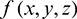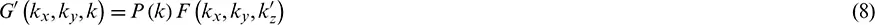We assumeConsidering the effect of signal bandwidth, the HFR imaging results can be approximately obtained by inverse Fourier transformation of the spectrum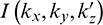: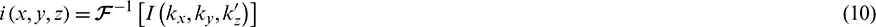where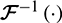is the inverse FFT (IFFT).

2.2 The Spectrum Migration

The imaging process of HFR imaging systems rely on FFT, which has an advantage of high computational efficiency. However, in practical applications, the utilization rate of the high frequency signals spectrum is usually low.

We analyze the imaging model corresponding to Eq. (10) whose spectrum range is determined by Eq. (7). For convenience and generality of the description, we use the two-dimensional imaging model to illustrate the spectrum migration. We assume that the object is a constant in the y direction, and thus ky = 0. We can then obtain the equation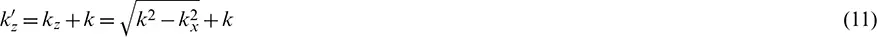During imaging, the ultrasonic propagation is in the direction of +z. Therefore, the values of kz should be greater than 0, but the values of kx can be positive or negative. The maximum value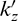will be obtained when k = 0. As a result, the range of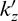is [0,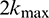]. The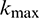and the maximum frequency component of echo signal (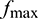) meets Eq. (3). We assume that the sampling depth is L and the sampling number is N. According to the property of the FFT operation, the sampling number in the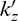direction is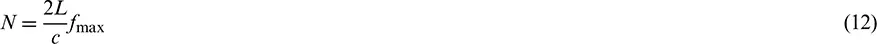According to the data provided by the Plane-wave Imaging Challenge in Medical Ultrasound (PICMUS) , the center frequency of the echo signal is about 4.5 MHz, and the maximum frequencyis around 6.8 MHz. We assume that the sound velocity c is 1540 m/s, and L is 180 mm. As a result, the sampling number N in the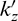direction is 1589. For convenience of FFT calculation, N is set to 2048. This number is not too large for a one-dimensional FFT but is relatively large for two-dimensional FFT. This leads to high requirements for data storage, transmission, and other hardware platforms. However, when N decreases, the effective high frequency part of the echo spectrum is not used leading to reduced utilization of the spectrum.

Due to the limited spectrum range of ultrasonic signals, the spectrum components in the low frequency (lower than fc1) and the high frequency (higher than fc2) are approximately zero (Fig. 1a). The effective spectrum components are mainly concentrated on the central frequency f0. Areas A and C of the imaging spectrum correspond to the low frequency (lower than fc1) and the high frequency (higher than fc2) in Fig. 1a, respectively, which has a relatively low spectrum energy and has little contribution to imaging. Area B in Fig. 1b plays an important role in imaging, and this corresponds to the spectrum around the central frequency f0; it ranges from fc1 to fc2. When the frequency of ultrasonic signal is high, the imaging spectrum distribution moves to region C in Fig. 1b. According to Eq. (12), the number of FFT points is increased, but the contribution to imaging is not great.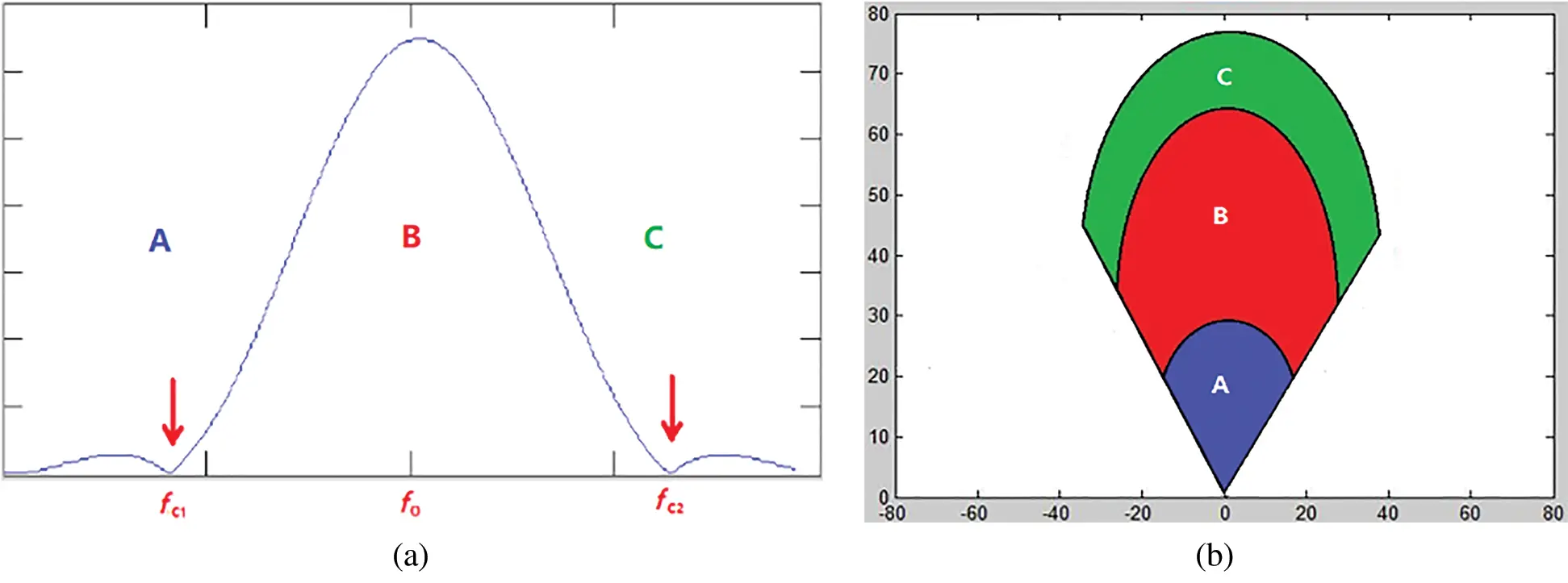Figure 1: Corresponding relationship between (a) ultrasonic signal spectrum and (b) imaging spectrum

Due to the limitations of the FFT points, the high frequency spectrum is not effectively utilized for imaging. Therefore, we adopt spectrum migration to move the imaging spectrum from high frequency to low frequency–-this approach not only fills the low frequency gap of the imaging spectrum but also moves the spectrum region of high frequency to the effective region required by FFT. Thus, the spectrum of the signal can be fully utilized. The wave number k is migrated, i.e.,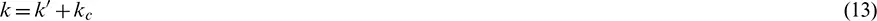Here, kc is the migration distance, and the relationship between kc and the frequency satisfies Eq. (3), i.e.,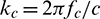. Term fc is the position of the frequency coordinate axis corresponding to the first minimum point on the left of the main lobe of the signal spectrum, and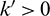.

After spectrum migration, the most concentrated part of the spectrum component of the signal, namely the main lobe, can be used to generate the spectrum of the image. Obviously, this migration maximizes the utilization rate of the spectrum of the signal.

Thus, according to Eq. (13), Eq. (9) can be rewritten as: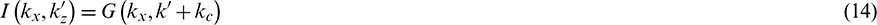where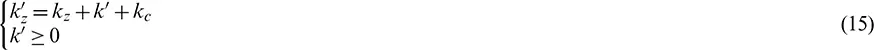The solution tocan be given by Eq. (15), and then the imagecan be obtained using IFFT.

3  Simulation and Experimental Results

3.1 Summary of Simulated and Experimental Data and Spectrum Analysis

The spectrum migration method was tested using data sets provided by the PICMUS organized by the IEEE International Ultrasonics Symposium (IUS). Simulated and experimental data sets contain 75 plane waves covering aangular span. Here, the echo data emitted by the plane wave acoustic field with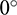were used. The parameters used in the simulation were completely the same with the same experimental settings. The sampling frequency was 20.832 MHz. The transducer was a 128-element linear array with a 0.27 mm width, 5.0 mm height, and 0.3 mm pitch. The transmitted signal (i.e., the excitation pulse imposing on the transducer) was two half-period sine waves with a center frequency of 5.208 MHz and a spectrum width of 67% of the center frequency. The simulated acoustic field and simulated data were acquired using the Filed II software developed by Professor Jensen of the Technical University of Denmark. It has a high accuracy in the ultrasonic acoustic field [14,15].

Fig. 2 is the waveform according to the simulated data. Fig. 2a is the waveform acquired by the first channel, and Fig. 2b is the data from the 400th to the 460th sampling points of the first channel. Figs. 2c and 2d is its spectrum. The effective spectrum distribution is 3 to 6.2 MHz.Figure 2: Waveform and signal spectrum of the first channel data in the simulation data

Fig. 3 is the waveform according to the experimental data in which Fig. 3a is the waveform collected by the first channel. This is experimental data, and there is echo data and noise interference when receiving. To clearly observe the spectrum distribution, a data segment with less noise interference is selected, i.e., points 1310th to 1340th as received by the first channel. The waveform of this segment is shown in Fig. 3b, and its spectrum is given in Figs. 3c and 3d. The distribution of the effective spectrum is basically consistent with the simulated data, and the distribution range is 3 to 6.8 MHz.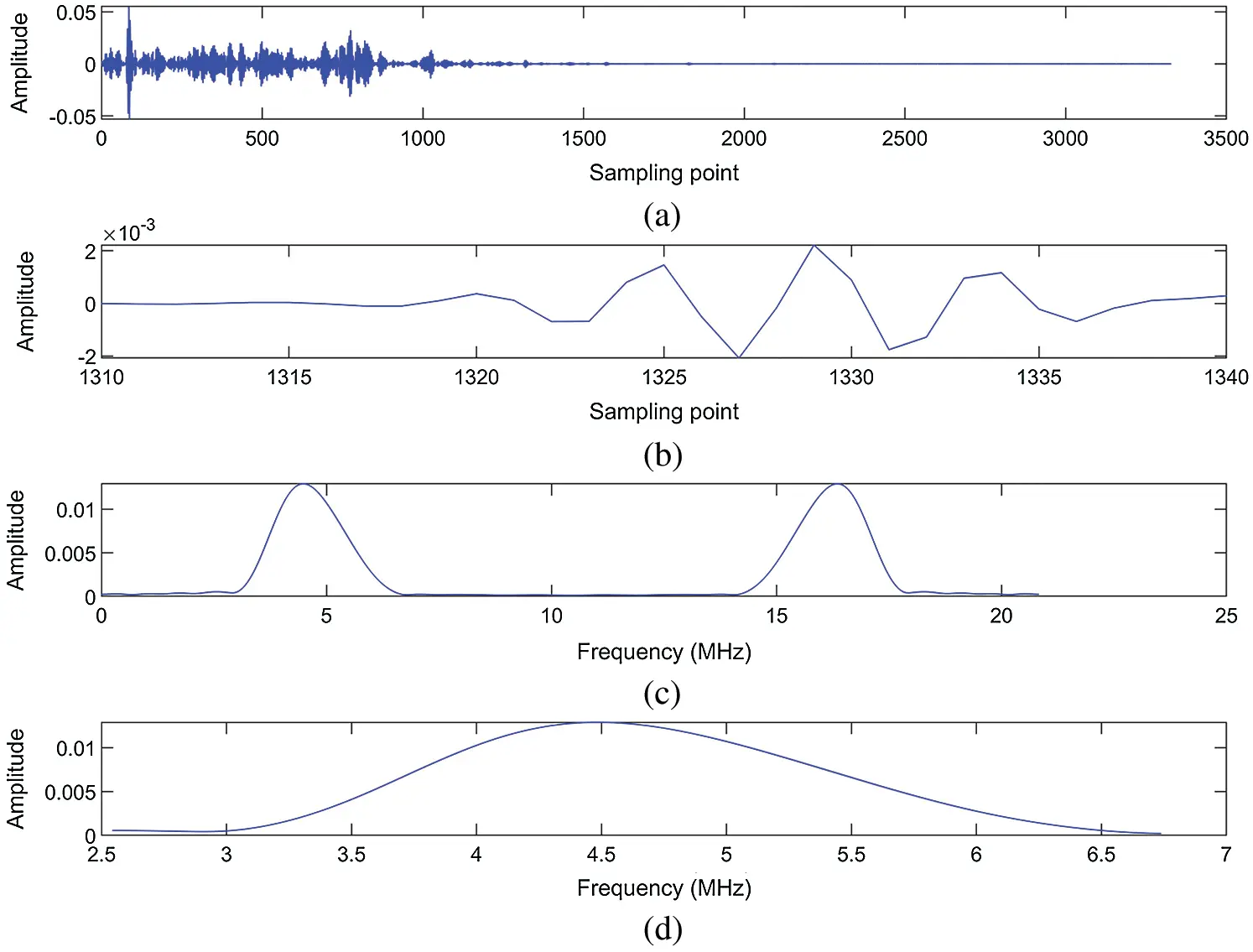Figure 3: Waveform and signal spectrum of the first channel data in the experimental data

3.2 Simulation Results

The simulated data set constitutes horizontally distributed and vertically distributed point targets. Fig. 4 displays the spectrum distribution of the simulated data over a 40 dB dynamic range, which is obtained by normalization and logarithmic compression.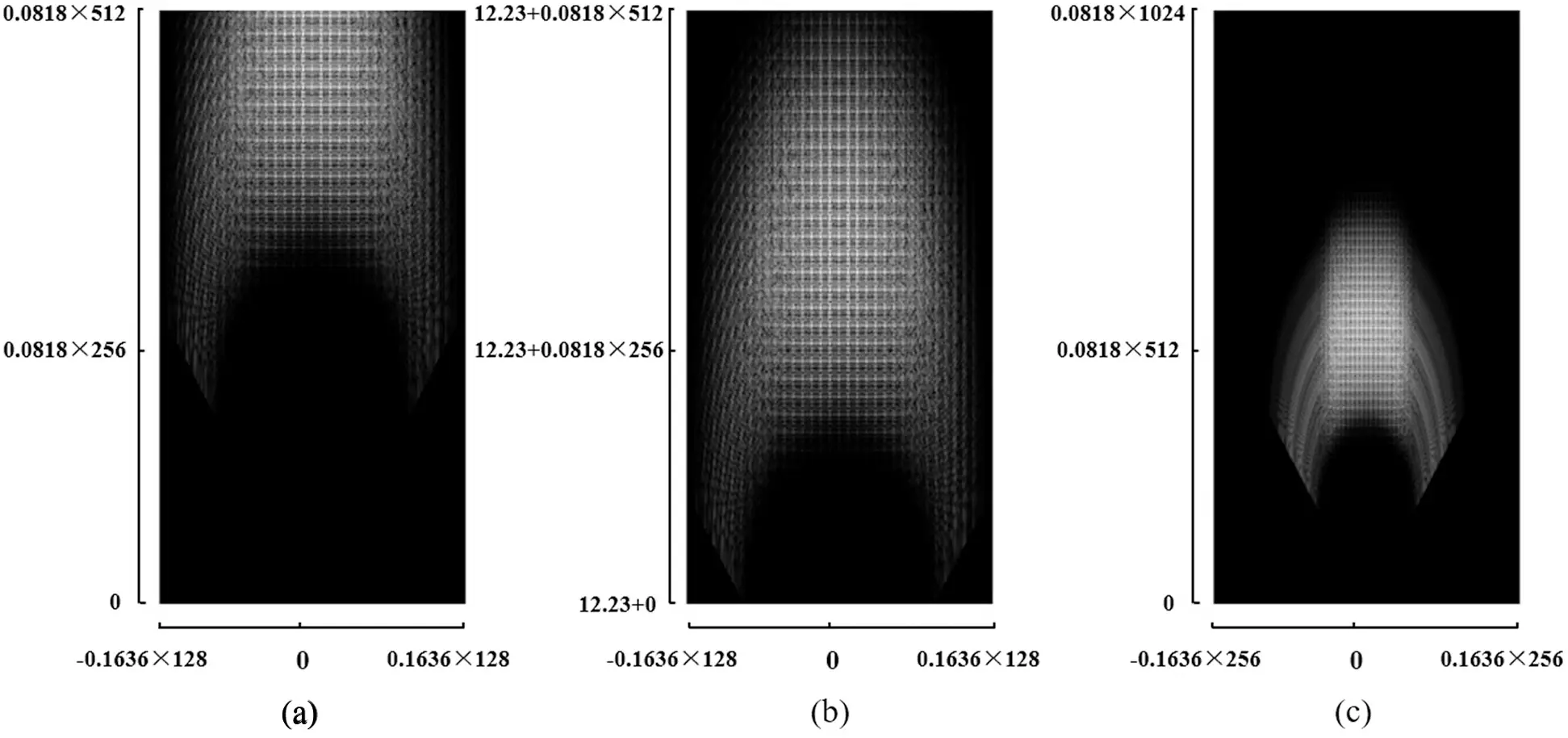Figure 4: Spectrum distribution of the simulated data obtained by different methods: (a) Fourier migration (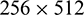FFT points), (b) spectrum migration, and (c) Fourier migration (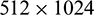FFT points)

The spectrum of the echo signal mainly ranges from 2 to 8 MHz; thus, the corresponding imaging spectrum range is 16.3–65.2 radians/mm. In the simulation, the image size is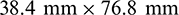, and the number of FFT points is. In this way, the unit in the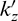direction is 0.082 radians/mm, and the value of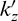corresponding to the 512th point is 42 radians/mm. As a result, the signal spectrum cannot be fully converted to the imaging spectrum using Fourier migration with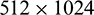FFT points. This phenomenon can be observed in the spectrum distribution in Fig. 4a. Fig. 4b shows the image spectrum obtained via the spectrum migration method, and the effective range of the spectrum is significantly increased. Fig. 4c shows the image spectrum obtained using the Fourier migration method with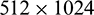FFT points. This spectrum distribution is essentially the spectrum of the signal converted to the image spectrum without any loss. The spectrum range in Fig. 4b basically contains the effective part of the spectrum shown in Fig. 4c.

Fig. 5a displays the image generated by the spectrum in Fig. 4a. Clearly, the side lobes are quite high, which degrade the resolution. vs. Fig. 5a however, the axial side lobes are effectively reduced in Fig. 5b due to the spectrum migration and the increased effective spectrum range. Fig. 5c shows the image generated using the spectrum in Fig. 4c. Although the complete signal spectrum is used for imaging, the image quality is basically the same as that in the spectrum migration method. The computational complexity of the Fourier migration withFFT points is four times larger than that of the spectrum migration method. Fig. 5d shows the DAS image. Compared with DAS, the proposed method shows fewer lateral sidelobes.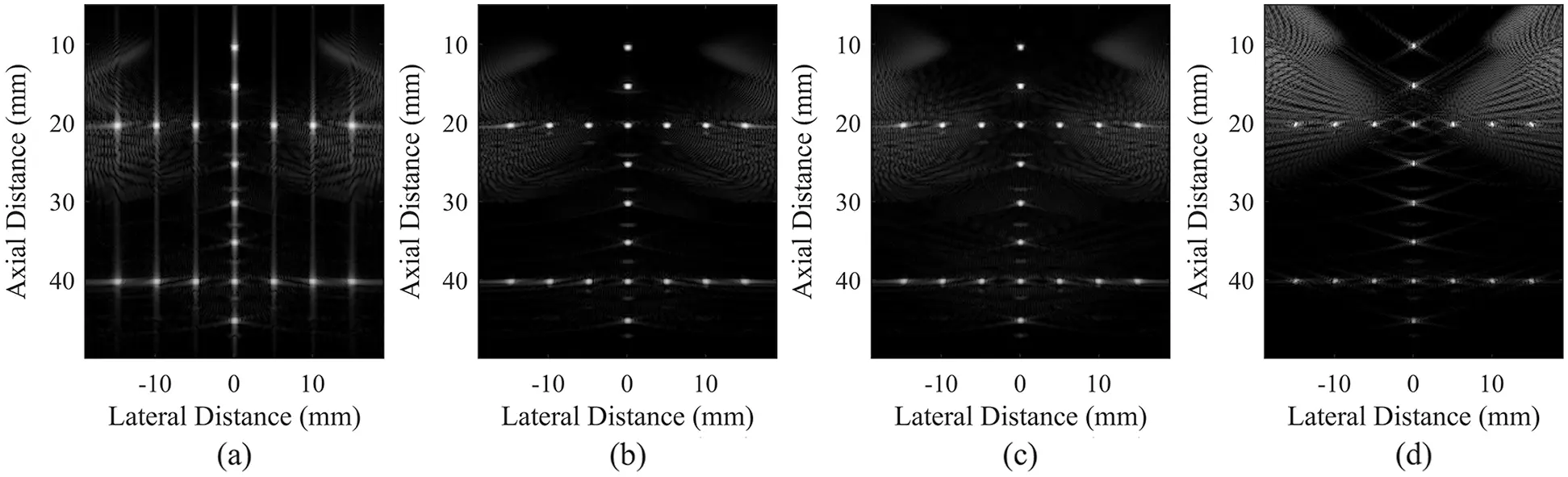Figure 5: Simulated point target imaging results obtained by different methods: (a) Fourier migration (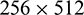FFT points), (b) spectrum migration, (c) Fourier migration (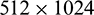FFT points), and (d) DAS

Figs. 6 and 7 present the axial variations of the vertically distributed points (x = 0 mm) and the lateral variations of horizontally distributed points (z = 20 mm), respectively. Fig. 6 shows that the spectrum migration method can effectively reduce the axial side lobes, which provides almost identical quality to that of the complete signal spectrum imaging on the distributions of point targets. Moreover, most of the axial side lobes of the Fourier migration with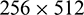FFT points are above −33 dB while the spectrum migration method almost completely suppresses the axial side lobe below −33 dB. The effective dynamic range and image resolution are used to quantitatively evaluate the imaging results. The effective dynamic range is defined as the difference between the lowest main lobe and the highest side lobe while the resolution is defined as the full width at half maximum of the point spread function (FWHM, −6 dB beam width of the main lobe). The effective dynamic range of Fig. 5a is about 11 dB while Figs. 5b and 5c obtained using the spectrum migration method and the complete spectrum imaging both have an effective dynamic range of about 17 dB. The average axial FWHM in Fig. 5a is 0.58 mm, and the average axial FWHMs of Figs. 5b and 5c are 0.38 and 0.39 mm, respectively. The average axial FHWMs of the image in Figs. 5a5c are 0.51, 0.51, and 0.48 mm, respectively. The data show that the spectrum migration method cannot significantly improve the lateral resolution but does improve the effective dynamic range and axial resolution. The proposed spectrum migration method increases the effective dynamic range by about 6 dB, and the average axial FWHM by 0.2 mm for simulated data vs. the Fourier migration.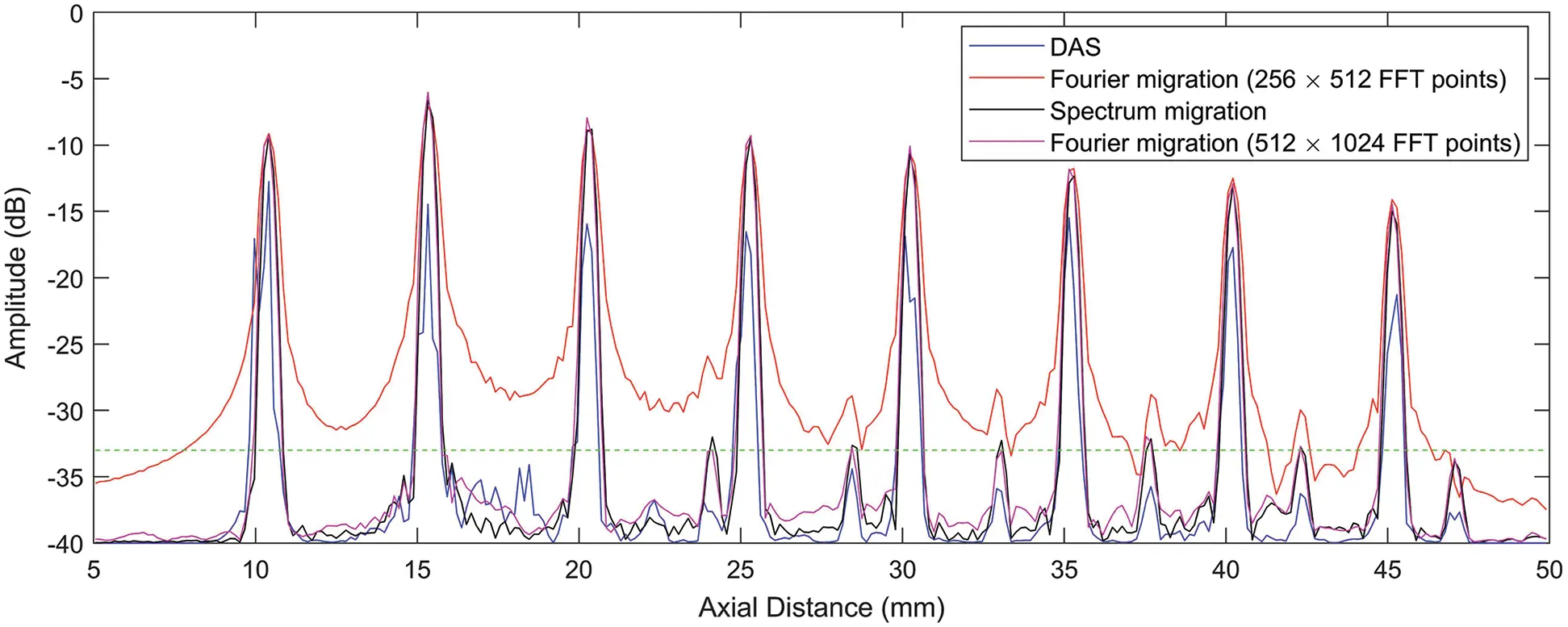Figure 6: Axial variation of the vertically distributed point (x = 0 mm) in simulation images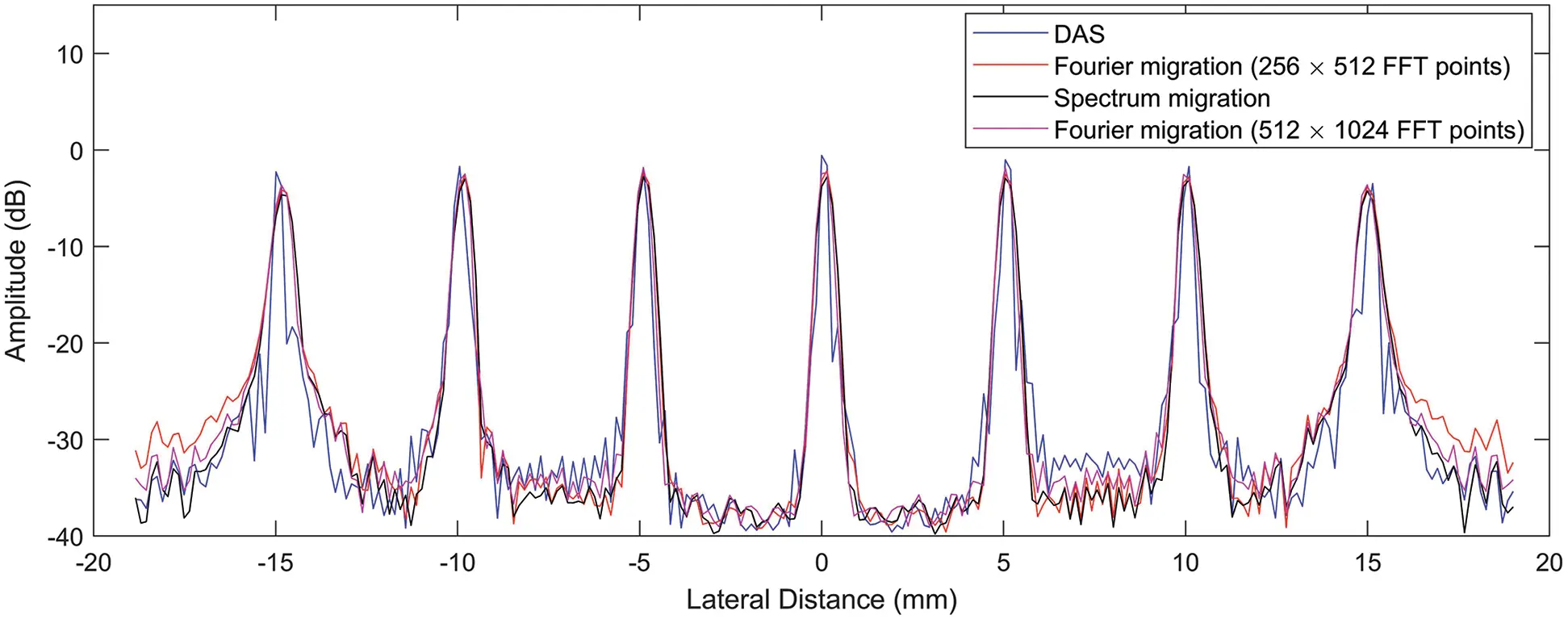Figure 7: The lateral variations of the horizontally distributed points (z = 20 mm) in simulation images

3.3 Experimental Results

There was massive cyst tissue and several point targets in standard ultrasonic phantom. The echo signal was acquired by a Vantage 256 transducer and an open multi-channel ultrasonic research platform produced by the Verasonics Corporation of the US.

Fig. 8 shows the spectrum of the experimental data, and the display dynamic range is also 40 dB. Like Fig. 4, the FFT points in Fig. 8a is also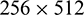. The spectrum of the echo signals cannot be completely included in the imaging spectrum. However, the effective range of the imaging spectrum is significantly increased via the spectrum migration method in Fig. 8b. In Fig. 8c, the FFT points are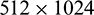, and the spectrum contains the complete spectrum range of the signal. By comparing Figs. 8b with 8c, the imaging spectrum obtained with the proposed spectrum migration method basically includes the main spectrum range of the signal.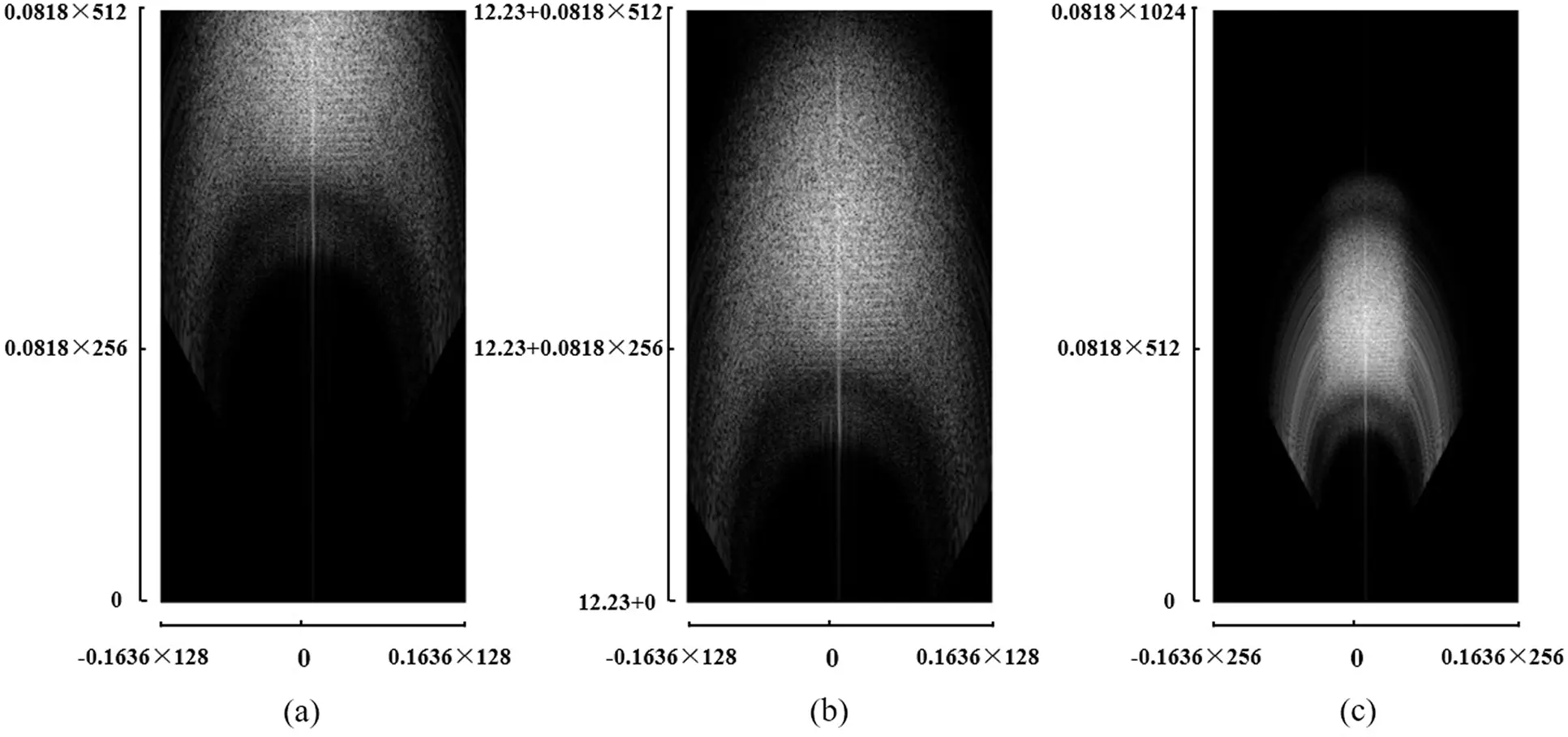Figure 8: Imaging spectrum of experimental data with different methods: (a) Fourier migration (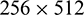FFT points), (b) spectrum migration, and (c) Fourier migration (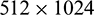FFT points)

The experimental results are given in Fig. 9 where each image in Figs. 9a9c corresponds to the image generated by the spectrum of Figs. 8a8c. Fig. 9b obtained by spectrum migration is basically the same as Fig. 9c obtained with the Fourier migration with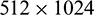FFT points. Compared to Fig. 9a, the axial side lobes are suppressed in Figs. 9b and 9c especially in the far-field region. The image of Fourier migration with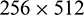FFT points shows obvious axial side lobes, which are effectively suppressed by the spectrum migration method. This can also be observed from Fig. 10: The spectrum migration method reduces the axial sidelobe by about 5 dB in the region at depths larger than 60 mm. From Figs. 9a and 9d, we can see that the spectrum migration method shows suppressed sidelobes and the more visible cyst, compared to DAS.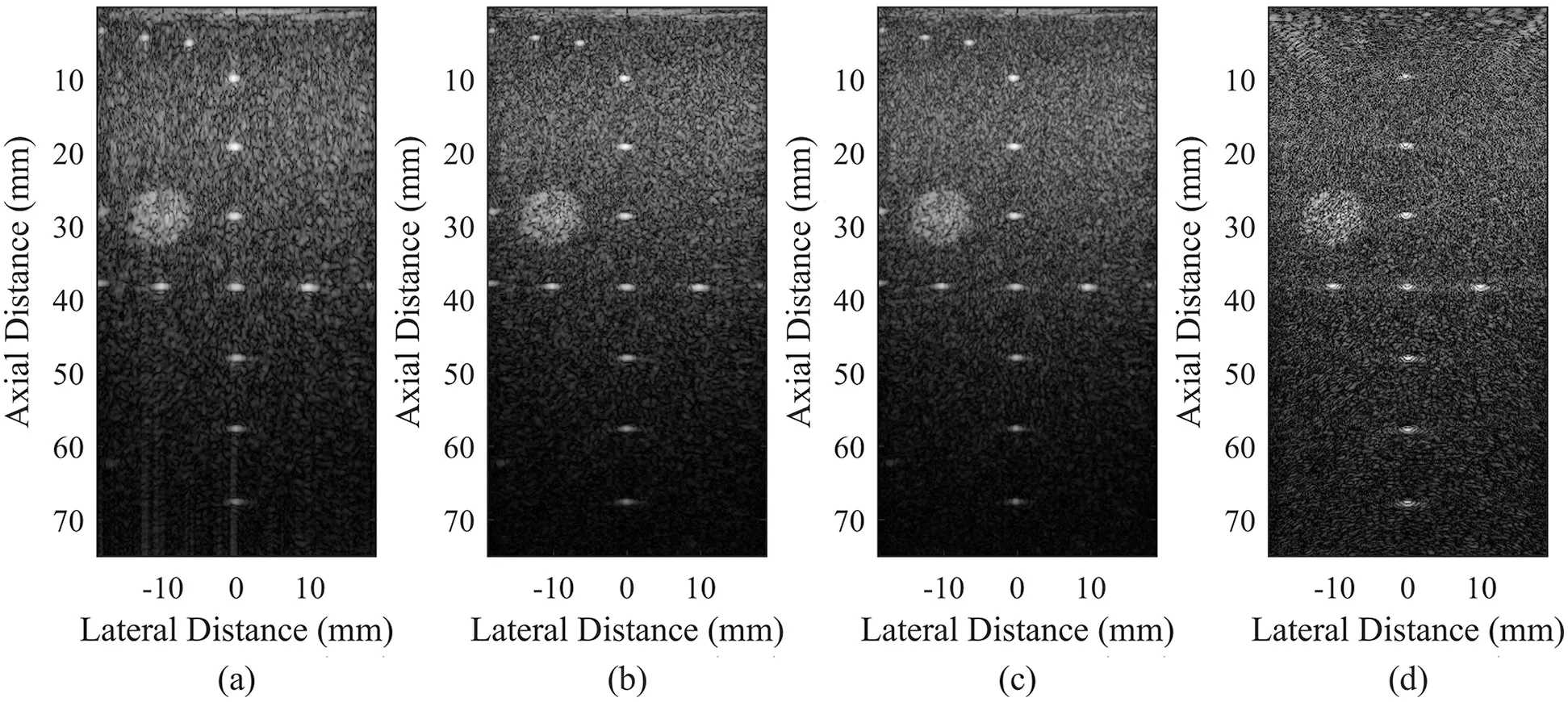Figure 9: Experimental point target imaging results obtained by different methods: (a) Fourier migration (FFT points), (b) spectrum migration, (c) Fourier migration (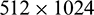FFT points), and (d) DAS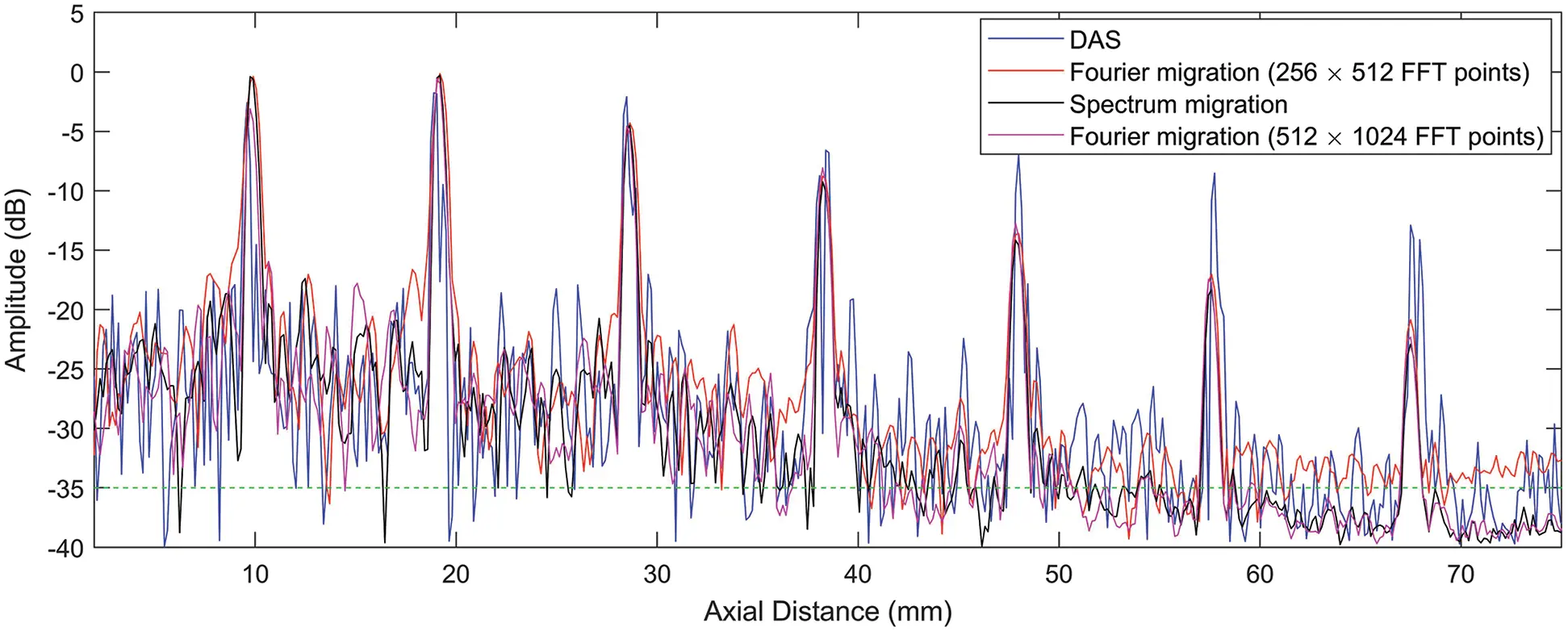Figure 10: The axial variations of the vertically distributed points in experimental images

Figs. 10 and 11 show the axial variations of vertically distributed points and the lateral variations of horizontally-distributed points, respectively. The mean lateral FWHM values of the point targets in experimental images obtained by the three methods are 1.22, 1.24, and 0.94 mm, and the mean axial FWHM values are 0.73, 0.61, and 0.61 mm for Fourier migration (FFT points), spectrum migration, and Fourier migration (FFT points), respectively. This is consistent with the simulation results. The spectrum migration method cannot improve the lateral resolution, but it significantly improves the axial resolution. In the experimental results, the axial mean FWHM improvement of the spectrum migration method is 0.12 mm compared to the Fourier migration with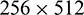FFT points.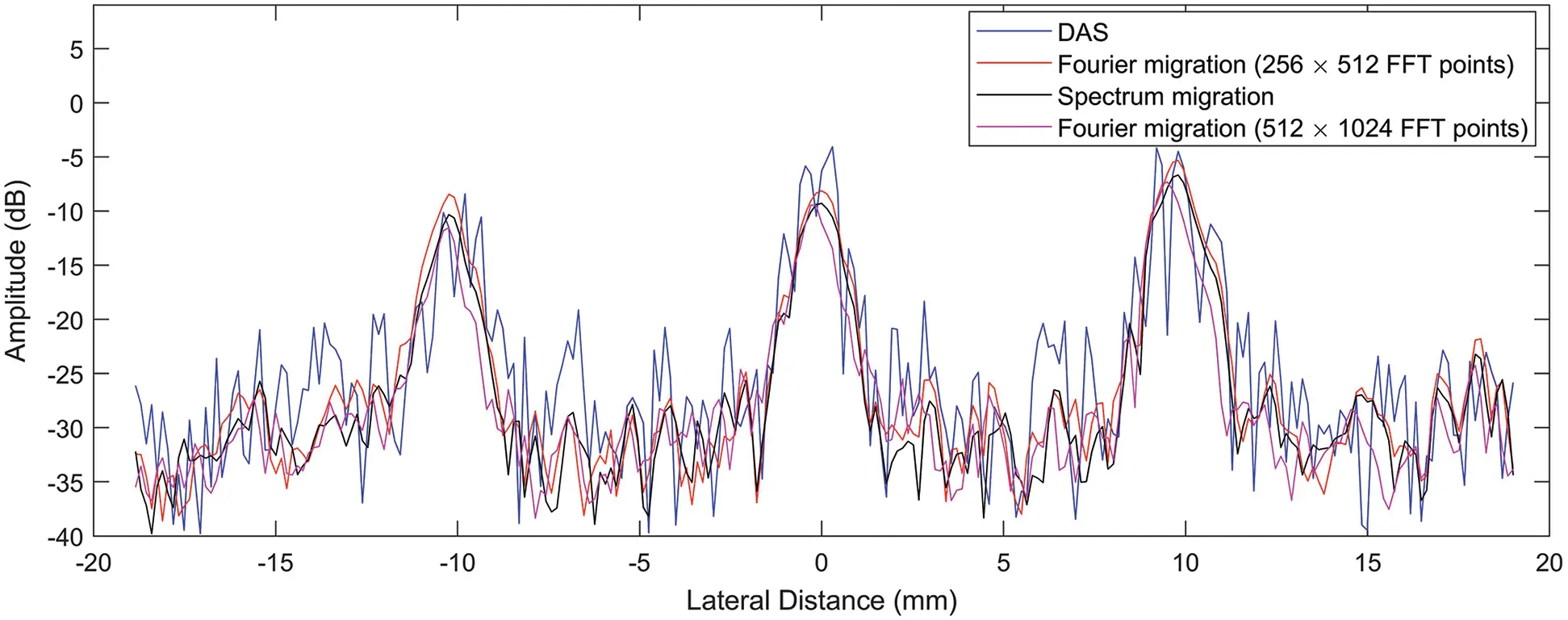Figure 11: The lateral variations of the horizontally distributed points in experimental images

4  Discussion

In Jensen’s study , the ultrasonic echo signal spectrum was converted to the imaging spectrum according to the constraint condition (Eq. (7)). This was called Fourier migration. However, Fourier migration requires a large number of FFT points so that the complete signal spectrum can be used for imaging–-particularly for echo signals with high frequency. Since the echo signals have a band-pass nature, the effective bandwidth and pulse width should be migrated to the imaging spectrum. However, there is no need to migrate the ineffective spectrum components that contribute little to imaging and usually contain noise. This will greatly reduce the number of FFT points and improve the efficiency of Fourier imaging while ensuring the imaging quality. Therefore, in this work, we proposed a spectrum migration method that only migrates the most concentrated part of the spectrum energy to the imaging spectrum region. Thus, it can reduce the FFT points and improve the efficiency of FFT. Moreover, the spectrum migration method utilizes the main components of signal spectrum to reconstruct images and thus obtain a high-quality image.

In both simulation and experiment, the transducer size is 38.4 mm, and the imaging depth is 76.8 mm. According to Eq. (12), when the speed of sound c and the imaging depth L are preset, the maximum frequency point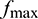of Fourier migration is determined by the number of FFT points N as shown in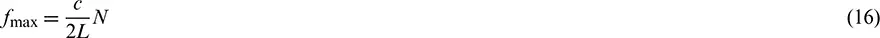When the FFT points N = 512 in the depth direction, the frequency range of the echo signal is about 5.1 MHz, and the effective spectrum range of the echo signal is between 3–6.5 MHz. If the spectrum migration method proposed in this paper is not adopted, then only about two-thirds of the signal spectrum is used to form the imaging spectrum while almost all the effective imaging spectrum can be utilized for imaging via spectrum migration. Section 3 shows that the starting frequency fc of the spectrum migration is 3 MHz. As a result, the spectrum of echo signals from 3–8.1 MHz is involved in the imaging spectrum. However, we can also choose the starting frequency of the spectrum migration as 2 MHz; correspondingly, the spectrum range of the echo signal is from 2–7.1 MHz for imaging. The following figures show the imaging results under these two conditions. Images obtained in these two conditions have the same image quality. This is because the echo signal spectrum has a limited bandwidth of about 3–6.5 MHz as shown in Figs. 12 and 13. These data indicate that the starting frequency fc can be set to values lower than 3 MHz for the spectrum migration method as long as the imaging spectrum range includes the effective spectrum.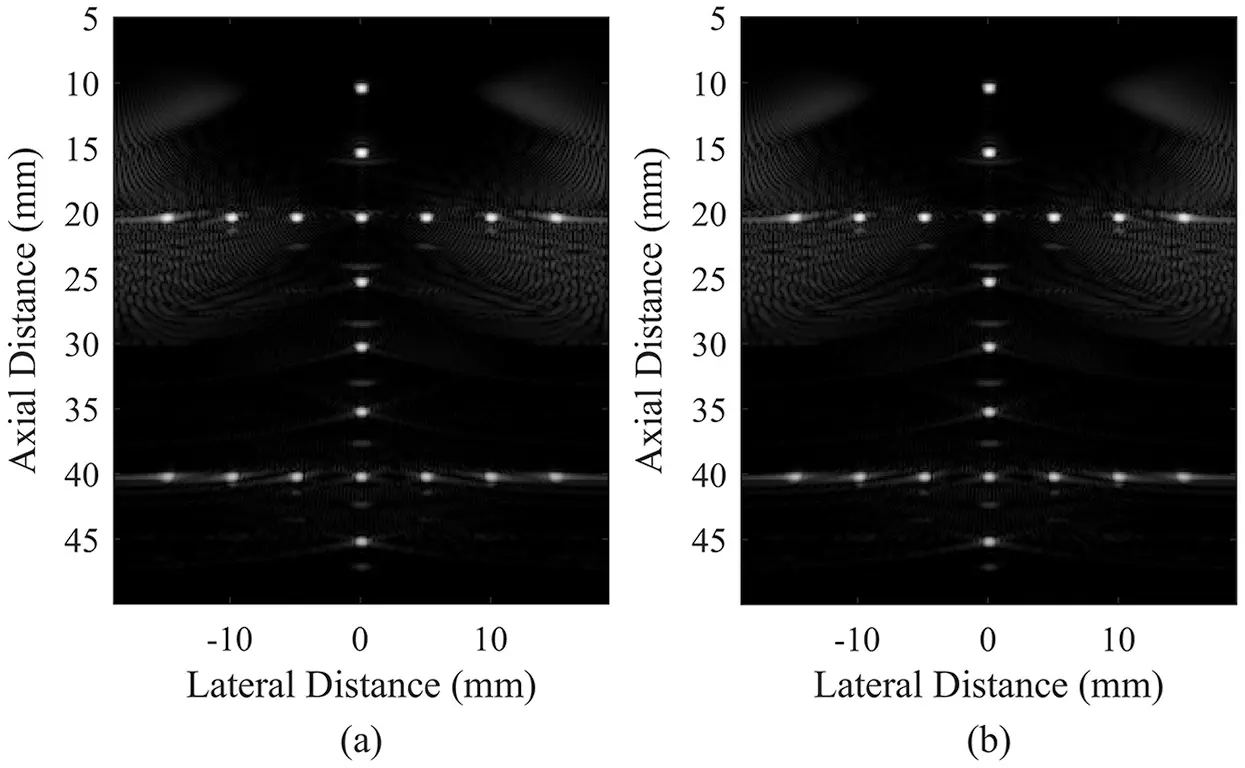Figure 12: Simulation imaging results obtained by the spectrum migration method: (a) fc = 3 MHz and (b) fc = 2 MHzFigure 13: Experimental imaging results obtained by the spectrum migration method: (a) fc = 3 MHz and (b) fc = 2 MHz

For two-dimensional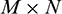data, the computational complexity of FFT operation is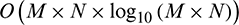. Figs. 4 and 9 show that both the number of FFT points of the Fourier migration and the spectrum migration method is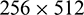, i.e., M = 256 and N = 512. The complete signal spectrum requires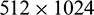FFT points, i.e., M = 512 and N = 1024. In other words, the computational complexity of the spectrum migration method is only about one-fourth that of the complete signal spectrum. Tab. 1 presents the contrast of different methods in spectrum utilization, image quality, and computational complexity. Combining the imaging results of simulation and experiment, we conclude that the spectrum migration method can not only achieve the same image quality as the complete spectrum imaging, but can also reduce the computational complexity by three-fold with significantly higher operational efficiency. In terms of computational efficiency, the graphics processing units (GPU) have been widely used to effectively reduce the computational time of algorithms . We will study to adopt a GPU-based parallel strategy for the spectrum migration method to accelerate the FFT process and further improve the imaging frame rate.

Table 1: Performance comparison of different methods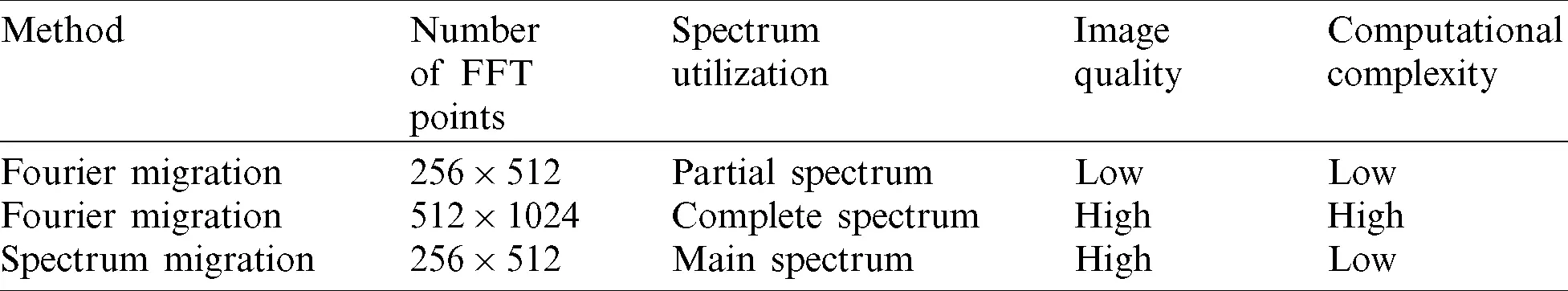5  Conclusion

This work presents an HFR imaging method using the spectrum migration technique. Considering that the echo signal is a bandwidth signal, the signal spectrum with a large energy contributes the most to the imaging spectrum while the part outside the effective spectrum with less energy has a smaller contribution to the imaging spectrum. Therefore, the proposed method ignores the less energy of the spectrum when converting the signal spectrum to the imaging spectrum; it only migrates the effective signal spectrum to the low-frequency region. This can effectively reduce the number of FFT transform points in the imaging process and improve the computational efficiency. Besides, the proposed method migrates the most concentrated part of the spectrum energy to the imaging spectrum region to guarantee high image quality. Results indicate that the spectrum migration method effectively improves the quality and computational efficiency of HFR imaging. vs. Fourier migration imaging with the same FFT points, the proposed spectrum migration method effectively suppresses the axial side lobes and improves the axial resolution thus improving the imaging quality. vs. complete spectrum imaging, this method not only achieves the same image quality but also reduces the computational complexity by three-fold. Therefore, the method proposed here is important in HFR imaging and has potential value in clinical applications.

Acknowledgement: The authors are grateful to the PICMUS organizers for sharing imaging data.

Funding Statement: This work is supported by National Natural Science Foundation of China, http://www.nsfc.gov.cn/. Peng H. received the project No. 62071165.

Conflicts of Interest: The authors declare that they have no conflicts of interest to report regarding the present study.

## References

1. Durnin, J. J. J. M., Miceli, J. J., & Eberly, J. H. (1987). Diffraction-free beams. Physical Review Letters, 58(15), 1499-1501. [Google Scholar] [CrossRef]
2. Ojeda-Castaneda, J., & Noyola-lglesias, A. (1990). Nondiffracting wavefields in grin and free-space. Microwave and Optical Technology Letters, 3(12), 430-433. [Google Scholar] [CrossRef]
3. Lu, J. Y. (1997). Designing limited diffraction beams. IEEE Transactions on Ultrasonics, Ferroelectrics, and Frequency Control, 44(1), 181-193. [Google Scholar] [CrossRef]
4. Lu, J. Y. (1997). Limited diffraction array beams. International Journal of Imaging Systems and Technology, 8(1), 126-136. [Google Scholar] [CrossRef]
5. Lu, J. Y. (1997). 2D and 3D high frame rate imaging with limited diffraction beams. IEEE Transactions on Ultrasonics, Ferroelectrics, and Frequency Control, 44(4), 839-856. [Google Scholar] [CrossRef]
6. Lu, J. Y. (2013). U.S. Patent No. 8,496,585. Washington, DC: U.S. Patent and Trademark Office.
7. Peng, H., Lu, J., & Han, X. (2006). High frame rate ultrasonic imaging system based on the angular spectrum principle. Ultrasonics, 44, e97-e99. [Google Scholar] [CrossRef]
8. Wei, Y. M., & Peng, H. (2014). Study on the transmission system of high frame rate ultrasonic imaging based on the non-diffraction wave. Acta Physica Sinica, 63(19), 198702. [Google Scholar]
9. Zhao, W., Feng, S., Wang, Y., Wang, Y., & Han, Z. (2020). A joint delay-and-sum and Fourier beamforming method for high frame rate ultrasound imaging. Computer Modeling in Engineering & Sciences, 123(1), 427-440. [Google Scholar] [CrossRef]
10. Cheng, J., & Lu, J. Y. (2006). Extended high-frame rate imaging method with limited-diffraction beams. IEEE Transactions on Ultrasonics, Ferroelectrics, and Frequency Control, 53(5), 880-899. [Google Scholar] [CrossRef]
11. Lu, J. Y., Cheng, J., & Wang, J. (2006). High frame rate imaging system for limited diffraction array beam imaging with square-wave aperture weightings. IEEE Transactions on Ultrasonics, Ferroelectrics, and Frequency Control, 53(10), 1796-1812. [Google Scholar] [CrossRef]
12. Chen, C., Hansen, H. H., Hendriks, G. A., Menssen, J., & Lu, J. Y. (2020). Point spread function formation in plane-wave imaging: A theoretical approximation in fourier migration. IEEE Transactions on Ultrasonics, Ferroelectrics, and Frequency Control, 67(2), 296-307. [Google Scholar] [CrossRef]
13. Liebgott, H., Rodriguez-Molares, A., Cervenansky, F., Jensen, J. A., Bernard, O. (2016). Plane-wave imaging challenge in medical ultrasound. IEEE International Ultrasonics Symposium, Tours, France, 1–4.
14. Jensen, J. A. (1996). Field: A program for simulating ultrasound systems. Medical & Biological Engineering & Computing, 34, 351-353. [Google Scholar] [CrossRef]
15. Jensen, J. A., & Svendsen, N. B. (1992). Calculation of pressure fields from arbitrarily shaped, apodized, and excited ultrasound transducers. IEEE Transactions on Ultrasonics, Ferroelectrics, and Frequency Control, 39(2), 262-267. [Google Scholar] [CrossRef]
16. Tang, J., Zheng, Y., Yang, C., Wang, W., & Luo, Y. (2020). Parallelized implementation of the finite particle method for explicit dynamics in GPU. Computer Modeling in Engineering & Sciences, 122(1), 5-31. [Google Scholar] [CrossRef]This work is licensed under a Creative Commons Attribution 4.0 International License, which permits unrestricted use, distribution, and reproduction in any medium, provided the original work is properly cited.